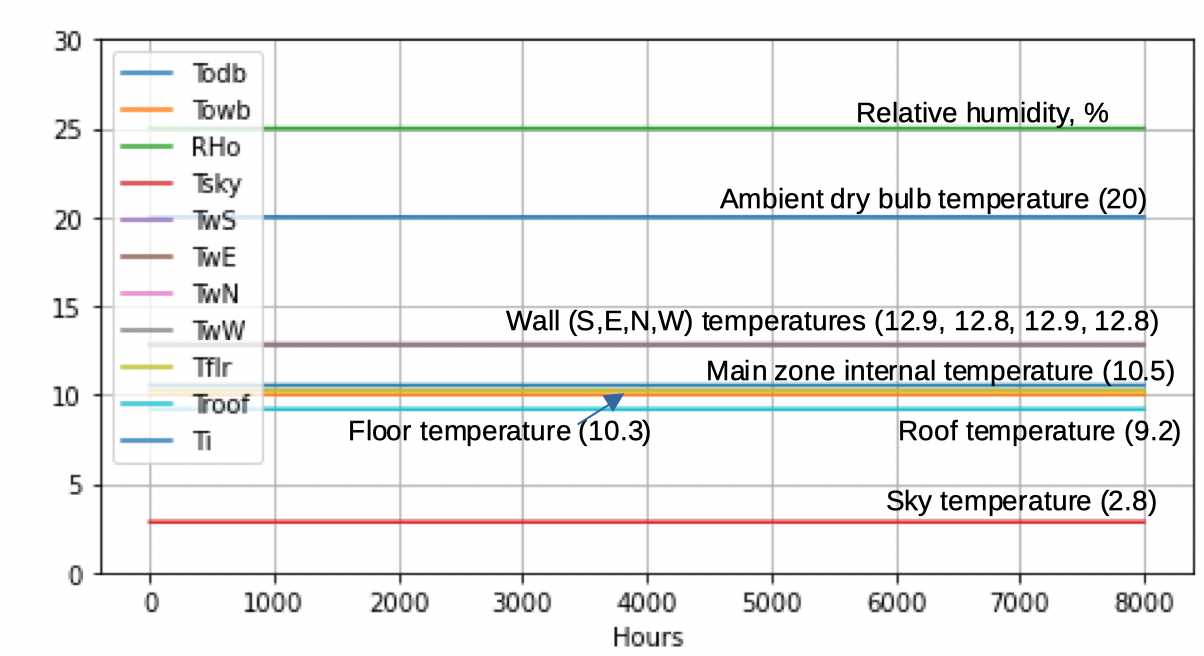Question-and-Answer Resource for the Building Energy Modeling Community
Get started with the Help page

Revision history [back]

With ericmartinpe's help as acknowledged above in my comments, I am now able to answer my own question.

The wind speed was the reason the internal temperature varied through the year even when the solar irradiance (all components) were set to zero and ambient temperature and humidity stayed constant through the year.

In my first posting, I had left the wind speed as it was in the original data file because I thought that the convection heat transfer would stop after the internal temperature became equal to the ambient dry bulb temperature. But I had forgottenabout the sky temperature. The sky teperature made the steel roof much colder than the ambient temperature and this caused convection heat transfer at rates varying with the wind speed as expected. When I set the wind speed to zero, then I get the following temperature traces:The above temperatures were generated by EnergyPlus using an artificial EPW file where the following environmental data were made constant through the year and day and night:

• DNI (Direct Normal Irradiance) = 0
• GHI (Global horizontal irradiance) = 0
• Ambient dry bulb temperature = 20 C
• Ambient relative humidity = 25%
• Atmospheric pressure = 100 kPa
• Dew point temperature = as computed for Tdb=20, RH=25% and P=100 kPa.
• Wind speed = 0 m/s
• Horizontal Infrared Radiation Intensity = 0

With ericmartinpe's help as acknowledged above in my comments, I am now able to answer my own question.

The wind speed was the reason the internal temperature varied through the year even when the solar irradiance (all components) were set to zero and ambient temperature and humidity stayed constant through the year.

In my first posting, I had left the wind speed as it was in the original data file because I thought that the convection heat transfer would stop after the internal temperature became equal to the ambient dry bulb temperature. But I had forgottenabout the sky temperature. The sky teperature made the steel roof much colder than the ambient temperature and this caused convection heat transfer at rates varying with the wind speed as expected. When I set the wind speed to zero, then I get the following temperature traces:The above temperatures (the numbers are temperatures in oC) were generated by EnergyPlus using an artificial EPW file where the following environmental data were made constant through the year and day and night:

• DNI (Direct Normal Irradiance) = 0
• GHI (Global horizontal irradiance) = 0
• Ambient dry bulb temperature = 20 C
• Ambient relative humidity = 25%
• Atmospheric pressure = 100 kPa
• Dew point temperature = as computed for Tdb=20, RH=25% and P=100 kPa.
• Wind speed = 0 m/s
• Horizontal Infrared Radiation Intensity = 0

With ericmartinpe's help as acknowledged above in my comments, I am now able to answer my own question.

The wind speed was the reason the internal temperature varied through the year even when the solar irradiance (all components) were set to zero and ambient temperature and humidity stayed constant through the year.

In my first posting, I had left the wind speed as it was in the original data file because I thought that the convection heat transfer would stop after the internal temperature became equal to the ambient dry bulb temperature. But I had forgottenabout the sky temperature. The sky teperature made the steel roof much colder than the ambient temperature and this caused convection heat transfer at rates varying with the wind speed as expected. When I set the wind speed to zero, then I get the following temperature traces:The above temperatures (the numbers are temperatures in oC) were generated by EnergyPlus using an artificial EPW file where the following environmental data were made constant through the year and day and night:

• DNI (Direct Normal Irradiance) = 0
• GHI (Global horizontal irradiance) = 0
• Ambient dry bulb temperature = 20 C
• Ambient relative humidity = 25%
• Atmospheric pressure = 100 kPa
• Dew point temperature = as computed for Tdb=20, RH=25% and P=100 kPa.
• Wind speed = 0 m/s
• Horizontal Infrared Radiation Intensity = 0